Theory and Modern Applications

# Synchronization stability of delayed discrete-time complex dynamical networks with randomly changing coupling strength

## Abstract

This paper addresses a delay-dependent synchronization stability problem for discrete-time complex dynamical networks with interval time-varying delays and randomly changing coupling strength. The randomly changing coupling strength is considered with the concept of binomial distribution. By constructing a suitable Lyapunov-Krasovskii functional and utilizing reciprocally convex approach and Finsler’s lemma, the proposed synchronization stability criteria for the networks are established in terms of linear matrix inequalities which can be easily solved by various effective optimization algorithms. The networks are represented by use of the Kronecker product technique. The effectiveness of the proposed methods will be verified via numerical examples.

## 1 Introduction

During the last few years, complex dynamic networks (CDNs), which is a set of interconnected nodes with specific dynamics, have received increasing attention from the real world such as the Internet, the World Wide Web, social networks, electrical power grids, global economic markets, and so on. Also, many models were proposed to describe various complex networks, small-world network and scale-free network, etc. For more details, see the literature . In the implementation of many practical CDNs, there exists time-delay because of the finite speed of information processing or amplifiers. It is well known that time-delay often causes undesirable dynamic behaviors such as oscillation and instability of the network. Therefore, various approaches to synchronization analysis for CDNs with time-delay have been investigated in the literature . By using network modeling with coupling delays, Li et al.  proposed, for the first time, the synchronization criteria for the CDNs with time-delay which were expressed in the form of linear matrix inequalities (LMIs). Koo et al.  presented a synchronization criterion for singular CDNs with time-varying delays. In , various synchronization problems are addressed for discrete-time CDNs with time-delay. In this regard, discrete-time modeling with time-delay plays an important role in many fields of CDNs. Moreover, to implement the network, the network uses digital computers (usually a microprocessor or microcontrollers) with the necessary input/output hardware. The fundamental character of the digital computer is that it computes answers at discrete steps.

On the other hand, in , the problems for various systems with randomly occurring delay, uncertainties and nonlinearities were considered. The randomly occurring considerations in these literature works are described by the Bernoulli distribution. Here, the Bernoulli distribution is recognized as the experiment for the combination of U identical subexperiments. For more details, let A be the elementary event having one of the two possible outcomes as its element. $\overline{A}$ is the only other possible elementary event. At this time, we shall repeat the basic experiments U times and determine the probability that A is observed exactly v times out of U trials. Such repeated experiments are called Bernoulli trials . Moveover, in , the stability of stochastic difference equations was addressed based on the Lyapunov functionals.

Regarding the CDNs, the coupling strength is the information of coupling strength between agents and a leader. Since an environmental change exists in practical networks, the change of coupling strength is a considerable factor affecting dynamics for the worse of the networks. At this point, the randomly changing coupling strength is being put to use in the problem of synchronization stability for CDNs. Moreover, to the best of authors’ knowledge, the synchronization analysis of CDNs with changing coupling strength has not been formulated yet.

Motivated by the results mentioned above, in this paper, a synchronization stability problem for discrete-time CDNs with interval time-varying delays and randomly changing coupling strength will be studied. This information is one of randomly occurring coupling strength with binomial distribution. Put simply, the first and simplest random variable is the Bernoulli random variable. Let X be a random variable that takes on only two possible numerical values, $X\left(\mathrm{\Omega }\right)=\left\{0,1\right\}$, where Ω represents the universal set consisting of the collection of all objects of interest in a particular context. Multiple independent Bernoulli random variables can be combined to construct more sophisticated random variables. Suppose X is the sum of w independent and identically distributed Bernoulli random variables. Then X is called a binomial random variable with parameters w, the number of trials, and p, the probability of success for each trial. Thus, the binomial distribution is a generalization of the Bernoulli distribution. Also, since delay-dependent analysis makes use of the information on the size of time delay, more attention has been paid to the delay-dependent analysis than to the delay-independent one . That is, the former is generally less conservative than the latter. Therefore, a great number of results on a delay-dependent stability condition for time-delay systems have been reported in the literature . So, by construction of a suitable Lyapunov-Krasovskii functional and utilization of a reciprocally convex approach , a synchronization stability problem for discrete-time CDNs with interval time-varying delays and randomly changing coupling strength is derived in terms of LMIs which can be solved efficiently by use of standard convex optimization algorithms such as interior-point methods . Moreover, the discrete-time CDNs are represented by use of the Kronecker product technique. Two numerical examples are included to show the effectiveness of the proposed methods.

Notation ${\mathbb{R}}^{n}$ is the n-dimensional Euclidean space, and ${\mathbb{R}}^{m×n}$ denotes the set of all $m×n$ real matrices. For real symmetric matrices X and Y, $X>Y$ (respectively, $X\ge Y$) means that the matrix $X-Y$ is positive definite (respectively, nonnegative). ${X}^{\perp }$ denotes the basis for the null-space of X. ${I}_{n}$, ${0}_{n}$, and ${0}_{m×n}$ denote $n×n$ identity matrix, $n×n$ and $m×n$ zero matrices, respectively. $\mathbb{E}\left\{\cdot \right\}$ stands for the mathematical expectation operator. $\parallel \cdot \parallel$ refers to the Euclidean vector norm or the induced matrix norm. $diag\left\{\cdots \right\}$ denotes the block diagonal matrix. represents the elements below the main diagonal of a symmetric matrix. ${X}_{\left[f\left(t\right)\right]}\in {\mathbb{R}}^{m×n}$ means that the elements of matrix ${X}_{\left[f\left(t\right)\right]}$ include the scalar value of $f\left(t\right)$.

## 2 Problem statements

Consider the following discrete-time CDNs with interval time-varying delays in the coupling term

${y}_{i}\left(k+1\right)=f\left({y}_{i}\left(k\right),{y}_{i}\left(k-h\left(k\right)\right)\right)+c\sum _{j=1}^{N}{g}_{ij}\mathrm{\Gamma }{y}_{j}\left(k-h\left(k\right)\right),\phantom{\rule{1em}{0ex}}i=1,2,\dots ,N.$
(1)

Here, N is the number of coupled nodes, n is the number of state of each node, ${y}_{i}\left(k\right)={\left[{y}_{i1}\left(k\right),{y}_{i2}\left(k\right),\dots ,{y}_{in}\left(k\right)\right]}^{T}\in {\mathbb{R}}^{n}$ is the state vector of the i th node. $f\left({y}_{i}\left(k\right)\right)=\left[f\left({y}_{i1}\left(k\right)\right),f\left({y}_{i2}\left(k\right)\right),\dots ,f\left({y}_{in}\left(k\right)\right)\right]\in {\mathbb{R}}^{n}$ is a continuous differentiable vector function. The constant $c>0$ is the coupling strength. $h\left(k\right)$ is an interval time-varying delay satisfying $0\le {h}_{m}\le h\left(k\right)\le {h}_{M}$, where ${h}_{m}$ and ${h}_{M}$ are known positive integers.

$\mathrm{\Gamma }={\left[{\gamma }_{ij}\right]}_{n×n}$ is the inner-coupling matrix of nodes, in which ${\gamma }_{ij}\ne 0$ means two coupled nodes are linked through their i th and j th state variables; otherwise, ${\gamma }_{ij}=0$. $G={\left[{g}_{ij}\right]}_{N×N}$ is the outer-coupling matrix of the network, in which ${g}_{ij}$ is defined as follows. If there is a connection between node i and node j ($j\ne i$), then ${g}_{ij}={g}_{ji}=1$; otherwise, ${g}_{ij}={g}_{ji}=0$ ($j\ne i$), and the diagonal elements of the matrix G are defined by

${g}_{ii}=-\sum _{j=1,i\ne j}^{N}{g}_{ij}=-\sum _{j=1,i\ne j}^{N}{g}_{ji},\phantom{\rule{1em}{0ex}}i=1,2,\dots ,N.$
(2)

In order to investigate the synchronization stability analysis for discrete-time CDNs with interval time-varying delays in the coupling term (1), we introduce the following definition and lemmas.

Definition 1 ()

The discrete-time delayed dynamical network (1) is said to achieve asymptotic synchronization if

(3)

where $s\left(k\right)\in {\mathbb{R}}^{n}$ is a solution of an isolated node, satisfying $s\left(k+1\right)=f\left(s\left(k\right),s\left(k-h\left(k\right)\right)\right)$.

Lemma 1 ()

Consider the network (1). Let $0={\lambda }_{1}>{\lambda }_{2}\ge \cdots \ge {\lambda }_{N}$ be the eigenvalues of the outer-coupling matrix G. If the following $N-1$ linear delayed difference equations are asymptotically stable about their zero solution

${x}_{l}\left(k+1\right)=J{x}_{l}\left(k\right)+{J}_{d}{x}_{l}\left(k-h\left(k\right)\right)+c{\lambda }_{l}\mathrm{\Gamma }{x}_{l}\left(k-h\left(k\right)\right),\phantom{\rule{1em}{0ex}}l=2,3,\dots ,N,$
(4)

where J and ${J}_{d}$ are the Jacobian of $f\left(x\left(k\right),x\left(k-h\left(k\right)\right)\right)$ at $s\left(k\right)$ and $s\left(k-h\left(k\right)\right)$, respectively. Then the synchronized states (3) are asymptotically stable.

For the convenience of synchronization analysis for the system (4), the following Kronecker product and its properties are used.

Lemma 2 (Kronecker product )

Let denote the notation of the Kronecker product. Then the following properties of the Kronecker product are easily established:

1. (i)

$\left(\alpha A\right)\otimes B=A\otimes \left(\alpha B\right)$,

2. (ii)

$\left(A+B\right)\otimes C=A\otimes C+B\otimes C$,

3. (iii)

$\left(A\otimes B\right)\left(C\otimes D\right)=\left(AC\right)\otimes \left(BD\right)$.

Let us define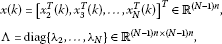where N is the number of agents.

Then the system (4) can be rewritten in the matrix form

$x\left(k+1\right)=\left({I}_{N-1}\otimes J\right)x\left(k\right)+\left({I}_{N-1}\otimes {J}_{d}\right)x\left(k-h\left(k\right)\right)+c\left(\mathrm{\Lambda }\otimes \mathrm{\Gamma }\right)x\left(k-h\left(k\right)\right).$
(5)

Moreover, it is assumed that the coupling strength has changed by the following assumption.

Assumption 1 The coupling strength is randomly changing. This means that, ${\rho }_{m}$ is a stochastic process representing the information changing process of coupling strength; that is, the transition of coupling strength is described by the following binomial probability:

$Pr\left\{{\rho }_{m}=m\right\}=\left(\genfrac{}{}{0}{}{l}{m}\right){\rho }_{0}^{m}{\left(1-{\rho }_{0}\right)}^{l-m},\phantom{\rule{1em}{0ex}}m=0,1,\dots ,l,$

where m is the number of changes, ${\rho }_{0}$ is the probability of change in one term and ${\rho }_{m}$ satisfies

$\mathbb{E}\left\{{\rho }_{m}\right\}=l{\rho }_{0}.$

With Assumption 1, a model of discrete-time CDNs with interval time-varying delays in the coupling term and the above assumptions is considered as

$x\left(k+1\right)=\left({I}_{N-1}\otimes J\right)x\left(k\right)+\left({I}_{N-1}\otimes {J}_{d}+{\rho }_{m}c\left(\mathrm{\Lambda }\otimes \mathrm{\Gamma }\right)\right)x\left(k-h\left(k\right)\right).$
(6)

Remark 1 As mentioned in Section 1, the Bernoulli random variable takes on only two possible numerical values, $X\left(\mathrm{\Omega }\right)=\left\{0,1\right\}$, where Ω represents the universal set consisting of the collection of all objects of interest in a particular context. However, with parameters w, the number of trials, and p, the probability of success for each trial, the binomial random variable X is the sum of w independent and identically distributed Bernoulli random variables. Thus, the former is more general than the latter. So, in this paper, the randomly changing coupling strength is considered with the concept of binomial distribution. In addition, the Bernoulli random variable has been used in the concept of randomly occurring which has various types such as randomly occurring delay, randomly occurring uncertainties, randomly occurring nonlinearities, and so on .

Remark 2 From Assumption 1, the coupling strength per discrete step is changed with the multiple of given strength, c, and the number of changes, m. Also, the probability of change is given as ${\rho }_{0}$. Based on the results mentioned above, for the concerned discrete-time CDNs (1), the coupling strength with the binomial random variable can be represented in the model of discrete-time CDNs with randomly changing coupling strength (6). It should be noted that this problem for the change of coupling strength has not been investigated yet.

The aim of this paper is to investigate the delay-dependent synchronization stability analysis for the system (6). In order to do this, we introduce the following definition and lemmas.

Lemma 3 For any constant matrix $0, integers ${h}_{m}$ and ${h}_{M}$ satisfying ${h}_{m}\le {h}_{M}$, and vector function $x:\left\{{h}_{m},{h}_{m}+1,\dots ,{h}_{M}\right\}\to {\mathbb{R}}^{n}$, the following inequality holds:

$-\left({h}_{M}-{h}_{m}+1\right)\sum _{s={h}_{m}}^{{h}_{M}}{x}^{T}\left(s\right)Mx\left(s\right)\le -{\left(\sum _{s={h}_{m}}^{{h}_{M}}x\left(s\right)\right)}^{T}M\left(\sum _{s={h}_{m}}^{{h}_{M}}x\left(s\right)\right).$
(7)

Proof From Lemma 1 in , the following inequality holds for ${h}_{m}\le s\le {h}_{M}$:

$\left[\begin{array}{cc}{x}^{T}\left(s\right)Mx\left(s\right)& {x}^{T}\left(s\right)\\ x\left(s\right)& {M}^{-1}\end{array}\right]\ge 0.$
(8)

Sum of the inequality (8) from ${h}_{m}$ to ${h}_{M}$ yields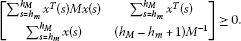(9)

Therefore, the inequality (9) is equivalent to the inequality (7) according to the Schur complement . □

Lemma 4 (Finsler’s lemma )

Let $\zeta \in {\mathbb{R}}^{n}$, $\mathrm{\Phi }={\mathrm{\Phi }}^{T}\in {\mathbb{R}}^{n×n}$, and $\mathrm{\Upsilon }\in {\mathbb{R}}^{m×n}$ such that $rank\left(\mathrm{\Upsilon }\right). The following statements are equivalent:

1. (i)

${\zeta }^{T}\mathrm{\Phi }\zeta <0$, $\mathrm{\forall }\mathrm{\Upsilon }\zeta =0$, $\zeta \ne 0$,

2. (ii)

${{\mathrm{\Upsilon }}^{\perp }}^{T}\mathrm{\Phi }{\mathrm{\Upsilon }}^{\perp }<0$,

3. (iii)

$\mathrm{\exists }F\in {\mathbb{R}}^{n×m}:\mathrm{\Phi }+F\mathrm{\Upsilon }+{\left(F\mathrm{\Upsilon }\right)}^{T}<0$.

## 3 Main results

In this section, we propose new synchronization criteria for the system (6). For the sake of simplicity on matrix representation, ${e}_{i}\in {\mathbb{R}}^{5\kappa ×\kappa }$, where $\kappa =\left(N-1\right)n$, are defined as block entry matrices, e.g., ${e}_{2}={\left[{0}_{\kappa },{I}_{\kappa },{0}_{\kappa },{0}_{\kappa },{0}_{\kappa }\right]}^{T}$. The notations of several matrices are defined as: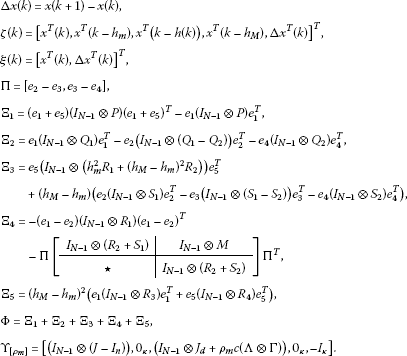(10)

Now, the following theorem is given for synchronization stability of the model of discrete-time CDNs with interval time-varying delays in the coupling term (6).

Theorem 1 For given positive integers ${h}_{m}$, ${h}_{M}$, l and positive scalars c, ${\rho }_{0}<1$, the system (6) is asymptotically synchronous for ${h}_{m}\le h\left(k\right)\le {h}_{M}$, if there exist positive matrices $P\in {\mathbb{R}}^{n×n}$, ${Q}_{i}\in {\mathbb{R}}^{n×n}$, ${R}_{j}\in {\mathbb{R}}^{n×n}$, any symmetric matrices ${S}_{i}\in {\mathbb{R}}^{n×n}$, where $i=1,2$ and $j=1,\dots ,4$, and any matrix $M\in {\mathbb{R}}^{n×n}$ satisfying the following LMIs: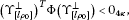(11)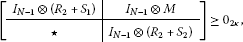(12)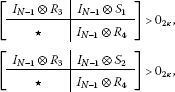(13)

where Φ and ${\mathrm{\Upsilon }}_{\left[{\rho }_{m}\right]}$ are defined in (10).

Proof Let us consider the following Lyapunov-Krasovskii functional candidate as

$V\left(k\right)={V}_{1}\left(k\right)+{V}_{2}\left(k\right)+{V}_{3}\left(k\right)+{V}_{4}\left(k\right),$
(14)

where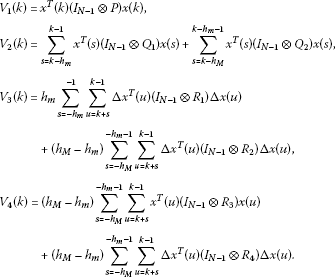The mathematical expectation of the $\mathrm{\Delta }{V}_{1}\left(k\right)$ and $\mathrm{\Delta }{V}_{2}\left(k\right)$ are calculated as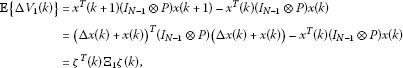(15)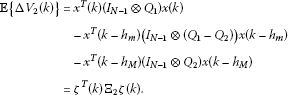(16)

By calculating the $\mathbb{E}\left\{\mathrm{\Delta }{V}_{3}\left(k\right)\right\}$, we get

$\begin{array}{rcl}\mathbb{E}\left\{\mathrm{\Delta }{V}_{3}\left(k\right)\right\}& =& \mathrm{\Delta }{x}^{T}\left(k\right)\left({I}_{N-1}\otimes \left({h}_{m}^{2}{R}_{1}+{\left({h}_{M}-{h}_{m}\right)}^{2}{R}_{2}\right)\right)\mathrm{\Delta }x\left(k\right)\\ -{h}_{m}\sum _{s=k-{h}_{m}}^{k-1}\mathrm{\Delta }{x}^{T}\left(s\right)\left({I}_{N-1}\otimes {R}_{1}\right)\mathrm{\Delta }x\left(s\right)\\ -\left({h}_{M}-{h}_{m}\right)\sum _{s=k-h\left(k\right)}^{k-{h}_{m}-1}\mathrm{\Delta }{x}^{T}\left(s\right)\left({I}_{N-1}\otimes {R}_{2}\right)\mathrm{\Delta }x\left(s\right)\\ -\left({h}_{M}-{h}_{m}\right)\sum _{s=k-{h}_{M}}^{k-h\left(k\right)-1}\mathrm{\Delta }{x}^{T}\left(s\right)\left({I}_{N-1}\otimes {R}_{2}\right)\mathrm{\Delta }x\left(s\right).\end{array}$
(17)

Inspired by the work of , the following two zero equalities hold with any symmetric matrices ${S}_{1}$ and ${S}_{2}$: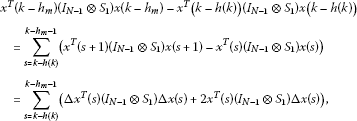(18)

and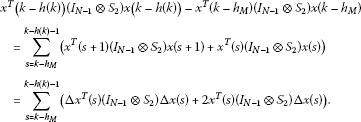(19)

Here, Eqs. (18) and (19) still hold even when we multiply both sides by $\left({h}_{M}-{h}_{m}\right)$. So, by adding the results into Eq. (17), we get

$\begin{array}{rcl}\mathbb{E}\left\{\mathrm{\Delta }{V}_{3}\left(k\right)\right\}& =& \mathrm{\Delta }{x}^{T}\left(k\right)\left({I}_{N-1}\otimes \left({h}_{m}^{2}{R}_{1}+{\left({h}_{M}-{h}_{m}\right)}^{2}{R}_{2}\right)\right)\mathrm{\Delta }x\left(k\right)\\ +\left({h}_{M}-{h}_{m}\right){x}^{T}\left(k-{h}_{m}\right)\left({I}_{N-1}\otimes {S}_{1}\right)x\left(k-{h}_{m}\right)\\ -\left({h}_{M}-{h}_{m}\right){x}^{T}\left(k-h\left(k\right)\right)\left({I}_{N-1}\otimes \left({S}_{1}-{S}_{2}\right)\right)x\left(k-h\left(k\right)\right)\\ -\left({h}_{M}-{h}_{m}\right){x}^{T}\left(k-{h}_{M}\right)\left({I}_{N-1}\otimes {S}_{2}\right)x\left(k-{h}_{M}\right)+\mathrm{\Sigma }+{\mathrm{\Theta }}_{1}\\ =& {\zeta }^{T}\left(k\right){\mathrm{\Xi }}_{3}\zeta \left(k\right)+\mathrm{\Sigma }+{\mathrm{\Theta }}_{1},\end{array}$
(20)

where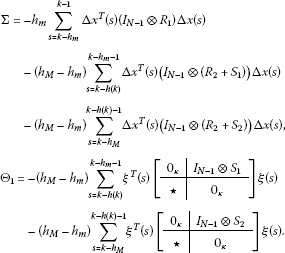By Lemma 3, the term Σ in (20) can be estimated as

$\begin{array}{rcl}\mathrm{\Sigma }& \le & -{\left(\sum _{s=k-{h}_{m}}^{k-1}\mathrm{\Delta }x\left(s\right)\right)}^{T}\left({I}_{N-1}\otimes {R}_{1}\right)\left(\sum _{s=k-{h}_{m}}^{k-1}\mathrm{\Delta }x\left(s\right)\right)\\ -{\left(\sum _{s=k-h\left(k\right)}^{k-{h}_{m}-1}\mathrm{\Delta }x\left(s\right)\right)}^{T}\left({I}_{N-1}\otimes \left({R}_{2}+{S}_{1}\right)\right)\left(\sum _{s=k-h\left(k\right)}^{k-{h}_{m}-1}\mathrm{\Delta }x\left(s\right)\right)\\ -{\left(\sum _{s=k-{h}_{M}}^{k-h\left(k\right)-1}\mathrm{\Delta }x\left(s\right)\right)}^{T}\left({I}_{N-1}\otimes \left({R}_{2}+{S}_{2}\right)\right)\left(\sum _{s=k-{h}_{M}}^{k-h\left(k\right)-1}\mathrm{\Delta }x\left(s\right)\right)\\ =& -{\zeta }^{T}\left(k\right)\left({e}_{1}-{e}_{2}\right)\left({I}_{N-1}\otimes {R}_{1}\right){\left({e}_{1}-{e}_{2}\right)}^{T}\zeta \left(k\right)\\ -{\zeta }^{T}\left(k\right)\mathrm{\Pi }\left[\begin{array}{cc}\frac{1}{1-\alpha \left(k\right)}\left({I}_{N-1}\otimes \left({R}_{2}+{S}_{1}\right)\right)& {0}_{\kappa }\\ \star & \frac{1}{\alpha \left(k\right)}\left({I}_{N-1}\otimes \left({R}_{2}+{S}_{2}\right)\right)\end{array}\right]{\mathrm{\Pi }}^{T}\zeta \left(k\right),\end{array}$
(21)

where $\alpha \left(k\right)=\left({h}_{M}-h\left(k\right)\right)/\left({h}_{M}-{h}_{m}\right)$.

Here, when ${h}_{m}, since $\alpha \left(k\right)$ satisfies $0<\alpha \left(t\right)<1$, by a reciprocally convex approach , the following inequality for any matrix M holds: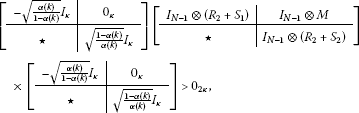which implies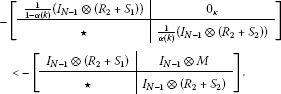(22)

Also, when $h\left(k\right)={h}_{m}$ or $h\left(k\right)={h}_{M}$, we get

$\begin{array}{rcl}\sum _{s=k-h\left(k\right)}^{k-{h}_{m}-1}\mathrm{\Delta }x\left(s\right)& =& \sum _{s=k-h\left(k\right)}^{k-{h}_{m}-1}\left(x\left(s+1\right)-x\left(s\right)\right)\\ =& x\left(k-{h}_{m}\right)-x\left(k-h\left(k\right)\right)\\ =& x\left(k-{h}_{m}\right)-x\left(k-{h}_{m}\right)={0}_{\kappa ×1}\end{array}$

or

$\begin{array}{rcl}\sum _{s=k-{h}_{M}}^{k-h\left(k\right)-1}\mathrm{\Delta }x\left(s\right)& =& \sum _{s=k-{h}_{M}}^{k-h\left(k\right)-1}\left(x\left(s+1\right)-x\left(s\right)\right)\\ =& x\left(k-h\left(k\right)\right)-x\left(k-{h}_{M}\right)\\ =& x\left(k-{h}_{M}\right)-x\left(k-{h}_{M}\right)={0}_{\kappa ×1},\end{array}$
(23)

respectively.

Thus, if the inequality (12) holds, then from Eqs. (22) and (23), the following inequality still holds:

$\begin{array}{rcl}\mathrm{\Sigma }& \le & -{\zeta }^{T}\left(k\right)\left({e}_{1}-{e}_{2}\right)\left({I}_{N-1}\otimes {R}_{1}\right){\left({e}_{1}-{e}_{2}\right)}^{T}\zeta \left(k\right)\\ -{\zeta }^{T}\left(k\right)\mathrm{\Pi }\left[\begin{array}{cc}\frac{1}{1-\alpha \left(k\right)}\left({I}_{N-1}\otimes \left({R}_{2}+{S}_{1}\right)\right)& {0}_{\kappa }\\ \star & \frac{1}{\alpha \left(k\right)}\left({I}_{N-1}\otimes \left({R}_{2}+{S}_{2}\right)\right)\end{array}\right]{\mathrm{\Pi }}^{T}\zeta \left(k\right)\\ \le & -{\zeta }^{T}\left(k\right)\left({e}_{1}-{e}_{2}\right)\left({I}_{N-1}\otimes {R}_{1}\right){\left({e}_{1}-{e}_{2}\right)}^{T}\zeta \left(k\right)\\ -{\zeta }^{T}\left(k\right)\mathrm{\Pi }\left[\begin{array}{cc}{I}_{N-1}\otimes \left({R}_{2}+{S}_{1}\right)& {I}_{N-1}\otimes M\\ \star & {I}_{N-1}\otimes \left({R}_{2}+{S}_{2}\right)\end{array}\right]{\mathrm{\Pi }}^{T}\zeta \left(k\right)\\ =& {\zeta }^{T}\left(k\right){\mathrm{\Xi }}_{4}\zeta \left(k\right),\end{array}$

which means

$\mathbb{E}\left\{\mathrm{\Delta }{V}_{3}\left(k\right)\right\}\le {\zeta }^{T}\left(k\right)\left({\mathrm{\Xi }}_{3}+{\mathrm{\Xi }}_{4}\right)\zeta \left(k\right)+{\mathrm{\Theta }}_{1}.$
(24)

Lastly, the $\mathbb{E}\left\{{V}_{4}\left(k\right)\right\}$ is calculated as

$\begin{array}{rcl}\mathbb{E}\left\{\mathrm{\Delta }{V}_{4}\left(k\right)\right\}& =& {\left({h}_{M}-{h}_{m}\right)}^{2}\left({x}^{T}\left(k\right)\left({I}_{N-1}\otimes {R}_{3}\right)x\left(k\right)+\mathrm{\Delta }{x}^{T}\left(k\right)\left({I}_{N-1}\otimes {R}_{4}\right)\mathrm{\Delta }x\left(k\right)\right)\\ -\left({h}_{M}-{h}_{m}\right)\sum _{s=k-h\left(k\right)}^{k-{h}_{m}-1}\left({x}^{T}\left(s\right)\left({I}_{N-1}\otimes {R}_{3}\right)x\left(s\right)+\mathrm{\Delta }{x}^{T}\left(s\right)\left({I}_{N-1}\otimes {R}_{4}\right)\mathrm{\Delta }x\left(s\right)\right)\\ -\left({h}_{M}-{h}_{m}\right)\sum _{s=k-{h}_{M}}^{k-h\left(k\right)-1}\left({x}^{T}\left(s\right)\left({I}_{N-1}\otimes {R}_{3}\right)x\left(s\right)+\mathrm{\Delta }{x}^{T}\left(s\right)\left({I}_{N-1}\otimes {R}_{4}\right)\mathrm{\Delta }x\left(s\right)\right)\\ =& {\zeta }^{T}\left(k\right){\mathrm{\Xi }}_{5}\zeta \left(k\right)+{\mathrm{\Theta }}_{2},\end{array}$
(25)

where

$\begin{array}{rcl}{\mathrm{\Theta }}_{2}& =& -\left({h}_{M}-{h}_{m}\right)\sum _{s=k-h\left(k\right)}^{k-{h}_{m}-1}{\xi }^{T}\left(s\right)\left[\begin{array}{cc}{I}_{N-1}\otimes {R}_{3}& {0}_{\kappa }\\ \star & {I}_{N-1}\otimes {R}_{4}\end{array}\right]\xi \left(s\right)\\ -\left({h}_{M}-{h}_{m}\right)\sum _{s=k-{h}_{M}}^{k-h\left(k\right)-1}{\xi }^{T}\left(s\right)\left[\begin{array}{cc}{I}_{N-1}\otimes {R}_{3}& {0}_{\kappa }\\ \star & {I}_{N-1}\otimes {R}_{4}\end{array}\right]\xi \left(s\right).\end{array}$

Furthermore, if the inequalities (13) hold, then the $\mathbb{E}\left\{\mathrm{\Delta }{V}_{3}\left(k\right)\right\}+\mathbb{E}\left\{\mathrm{\Delta }{V}_{4}\left(k\right)\right\}$ has an upper bound as follows:

$\begin{array}{rcl}\mathbb{E}\left\{\mathrm{\Delta }{V}_{3}\left(k\right)\right\}+\mathbb{E}\left\{\mathrm{\Delta }{V}_{4}\left(k\right)\right\}& \le & {\zeta }^{T}\left(k\right)\left({\mathrm{\Xi }}_{3}+{\mathrm{\Xi }}_{4}+{\mathrm{\Xi }}_{5}\right)\zeta \left(k\right)+\left({\mathrm{\Theta }}_{1}+{\mathrm{\Theta }}_{2}\right)\\ =& {\zeta }^{T}\left(k\right)\left({\mathrm{\Xi }}_{3}+{\mathrm{\Xi }}_{4}+{\mathrm{\Xi }}_{5}\right)\zeta \left(k\right)\\ -\left({h}_{M}-{h}_{m}\right)\sum _{s=k-h\left(k\right)}^{k-{h}_{m}-1}{\xi }^{T}\left(s\right)\left[\begin{array}{cc}{I}_{N-1}\otimes {R}_{3}& {I}_{N-1}\otimes {S}_{1}\\ \star & {I}_{N-1}\otimes {R}_{4}\end{array}\right]\xi \left(s\right)\\ -\left({h}_{M}-{h}_{m}\right)\sum _{s=k-{h}_{M}}^{k-h\left(k\right)-1}{\xi }^{T}\left(s\right)\left[\begin{array}{cc}{I}_{N-1}\otimes {R}_{3}& {I}_{N-1}\otimes {S}_{2}\\ \star & {I}_{N-1}\otimes {R}_{4}\end{array}\right]\xi \left(s\right)\\ \le & {\zeta }^{T}\left(k\right)\left({\mathrm{\Xi }}_{3}+{\mathrm{\Xi }}_{4}+{\mathrm{\Xi }}_{5}\right)\zeta \left(k\right).\end{array}$
(26)

Therefore, from Eqs. (15)-(26) and by application of the S-procedure , the mathematical expectation on $\mathrm{\Delta }V\left(k\right)$ has a new upper bound as

$\mathbb{E}\left\{\mathrm{\Delta }V\left(k\right)\right\}\le \mathbb{E}\left\{{\zeta }^{T}\left(k\right)\underset{\mathrm{\Phi }}{\underset{⏟}{\left({\mathrm{\Xi }}_{1}+{\mathrm{\Xi }}_{2}+{\mathrm{\Xi }}_{3}+{\mathrm{\Xi }}_{4}+{\mathrm{\Xi }}_{5}\right)}}\zeta \left(k\right)\right\}.$
(27)

Also, the system (6) with the augmented vector $\zeta \left(k\right)$ can be rewritten as

$\mathbb{E}\left\{{\mathrm{\Upsilon }}_{\left[{\rho }_{m}\right]}\zeta \left(k\right)\right\}={0}_{\kappa ×1},$
(28)

where ${\mathrm{\Upsilon }}_{\left[{\rho }_{m}\right]}$ is defined in (10).

Then a delay-dependent stability condition for the system (6) is

$\mathbb{E}\left\{{\zeta }^{T}\left(k\right)\mathrm{\Phi }\zeta \left(k\right)\right\}<0$
(29)

subject to

$\mathbb{E}\left\{{\mathrm{\Upsilon }}_{\left[{\rho }_{m}\right]}\zeta \left(k\right)\right\}={0}_{\kappa ×1}.$

From Lemma 4(iii) and Assumption 1, the inequality (29) is equivalent to the following condition:

$\begin{array}{rcl}\mathbb{E}\left\{\mathrm{\Phi }+F{\mathrm{\Upsilon }}_{\left[{\rho }_{m}\right]}+{\left(F{\mathrm{\Upsilon }}_{\left[{\rho }_{m}\right]}\right)}^{T}\right\}& =& \underset{{\stackrel{˜}{\mathrm{\Phi }}}_{\left[l{\rho }_{0}\right]}}{\underset{⏟}{\mathrm{\Phi }+F{\mathrm{\Upsilon }}_{\left[l{\rho }_{0}\right]}+{\left(F{\mathrm{\Upsilon }}_{\left[l{\rho }_{0}\right]}\right)}^{T}}}\\ <& {0}_{5\kappa },\end{array}$
(30)

where F is any matrix with appropriate dimension.

Here, by utilizing Lemma 4(ii), the condition (30) is equivalent to the following inequality:

${\left({\mathrm{\Upsilon }}_{\left[l{\rho }_{0}\right]}^{\perp }\right)}^{T}\mathrm{\Phi }\left({\mathrm{\Upsilon }}_{\left[l{\rho }_{0}\right]}^{\perp }\right)<{0}_{4\kappa }.$
(31)

From the inequality (31), if the LMIs (11)-(13) are satisfied, then the synchronization stability condition (29) holds by Definition 1. This completes our proof. □

As a special case, consider the following discrete-time CDNs with only interval time-varying delays in nodes and randomly changing coupling strength:

${y}_{i}\left(k+1\right)=f\left({y}_{i}\left(k\right),{y}_{i}\left(k-h\left(k\right)\right)\right)+{\rho }_{m}c\sum _{j=1}^{N}{g}_{ij}\mathrm{\Gamma }{y}_{j}\left(k\right),\phantom{\rule{1em}{0ex}}i=1,2,\dots ,N.$
(32)

By use of the similar method in the driven procedure of the model (6), a model of discrete-time CDNs (32) can be obtained as

$x\left(k+1\right)=\left({I}_{N-1}\otimes J+{\rho }_{m}c\left(\mathrm{\Lambda }\otimes \mathrm{\Gamma }\right)\right)x\left(k\right)+\left({I}_{N-1}\otimes {J}_{d}\right)x\left(k-h\left(k\right)\right).$
(33)

The following is given for synchronization stability of the model of discrete-time CDNs with only interval time-varying delays in nodes (33).

Theorem 2 For given positive integers ${h}_{m}$, ${h}_{M}$, l, and positive scalars c, ${\rho }_{0}<1$, the system (33) is asymptotically synchronous for ${h}_{m}\le h\left(k\right)\le {h}_{M}$, if there exist positive matrices $P\in {\mathbb{R}}^{n×n}$, ${Q}_{i}\in {\mathbb{R}}^{n×n}$, ${R}_{j}\in {\mathbb{R}}^{n×n}$, any symmetric matrices ${S}_{i}\in {\mathbb{R}}^{n×n}$, $i=1,2$, $j=1,\dots ,4$, and any matrix $M\in {\mathbb{R}}^{n×n}$ satisfying the following LMIs with (12) and (13):

${\left({\stackrel{ˆ}{\mathrm{\Upsilon }}}_{\left[l{\rho }_{0}\right]}^{\perp }\right)}^{T}\mathrm{\Phi }\left({\stackrel{ˆ}{\mathrm{\Upsilon }}}_{\left[l{\rho }_{0}\right]}^{\perp }\right)<{0}_{4\kappa }.$
(34)

Proof The above criterion is derived in the similar method as the proof of Theorem 1, instead of the matrix ${\mathrm{\Upsilon }}_{\left[{\rho }_{m}\right]}$, using the following matrix:

${\stackrel{ˆ}{\mathrm{\Upsilon }}}_{\left[{\rho }_{m}\right]}=\left[\left({I}_{N-1}\otimes \left(J-{I}_{n}\right)+{\rho }_{m}c\left(\mathrm{\Lambda }\otimes \mathrm{\Gamma }\right)\right),{0}_{\kappa },\left({I}_{N-1}\otimes {J}_{d}\right),{0}_{\kappa },-{I}_{\kappa }\right].$

The other procedure is straightforward from the proof of Theorem 1, so it is omitted. □

Remark 3 The systems (6) and (33) with randomly changing coupling strength and the switched systems  are similar in the concept of changing parameters. In , the various problems for the switched neural networks with time-invariant delay were addressed. However, since time delay has not only a fixed value in a practical system , the concerned systems with interval time-varying delays were considered in this paper. Moreover, the changing information of a parameter was considered with the probabilistic rule; that is, the Bernoulli sequence.

## 4 Numerical examples

In this section, we provide two numerical examples to show the effectiveness of the presented stability criteria in this paper.

Example 1 Consider the following 2-order system with the structure in Figure 1 and the inner-coupling matrix $\mathrm{\Gamma }=0.01{I}_{n}$: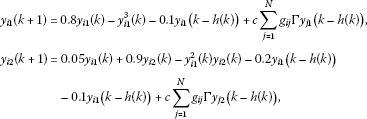(35)

which is asymptotically stable at the equilibrium point $s\left(k\right)=0$ and $s\left(k-h\left(k\right)\right)=0$. To analyse the synchronization stability for randomly changing coupling strength, the $N-1$ linear delayed difference equations (6) are

$x\left(k+1\right)=\left({I}_{N-1}\otimes J\right)x\left(k\right)+\left({I}_{N-1}\otimes {J}_{d}+{\rho }_{m}c\left(\mathrm{\Lambda }\otimes \mathrm{\Gamma }\right)\right)x\left(k-h\left(k\right)\right)$
(36)

with the Jacobian matrices

$J=\left[\begin{array}{cc}0.8& 0\\ 0.05& 0.9\end{array}\right],\phantom{\rule{2em}{0ex}}{J}_{d}=\left[\begin{array}{cc}-0.1& 0\\ -0.2& -0.1\end{array}\right].$

From Figure 1, the outer-coupling matrices are considered as two cases.

• Case 1:

$G=\left[\begin{array}{ccccc}-2& 1& 0& 0& 1\\ 1& -3& 1& 1& 0\\ 0& 1& -2& 1& 0\\ 0& 1& 1& -3& 1\\ 1& 0& 0& 1& -2\end{array}\right],$
• Case 2:

$G=\left[\begin{array}{cccccccccc}-4& 1& 0& 0& 1& 1& 1& 0& 0& 0\\ 1& -3& 0& 0& 0& 0& 0& 1& 1& 0\\ 0& 0& -1& 0& 0& 0& 0& 1& 0& 0\\ 0& 0& 0& -2& 0& 1& 0& 1& 0& 0\\ 1& 0& 0& 0& -2& 0& 0& 0& 1& 0\\ 1& 0& 0& 1& 0& -2& 0& 0& 0& 0\\ 1& 0& 0& 0& 0& 0& -3& 0& 1& 1\\ 0& 1& 1& 1& 0& 0& 0& -3& 0& 0\\ 0& 1& 0& 0& 1& 0& 1& 0& -4& 1\\ 0& 0& 0& 0& 0& 0& 1& 0& 1& -2\end{array}\right].$

Moreover, from Case 1,

$\mathrm{\Lambda }=diag\left\{-4.6180,-3.6180,-2.3820,-1.3820\right\}$

and from Case 2,

$\begin{array}{rcl}\mathrm{\Lambda }& =& diag\left\{-6.0399,-4.5664,-4.2269,-3.4438,-2.5493,\\ -2.1762,-1.6301,-0.9225,-0.4448\right\}.\end{array}$

The result of the maximum bound of time-delay with fixed $c=0.5$, $l=10$, ${\rho }_{0}=0.7$, ${h}_{m}=1$ and G in Case 1 provided by Theorem 1 is 5. For Case 1, Figure 2 shows the simulation results for the state trajectories of the network (36) with $h\left(k\right)=4|sin\left(k\pi /2\right)|+1$. When the coupling strength is changeless, the size of overshoot shown in Figure 2(b) is smaller than the one shown in Figure 2(a) at time . Here, if we consider the setting time and rise time on the basis of Figure 2(b), which are the results of an ideal model for CDNs without the changing coupling strength, we know the effect of the change of coupling strength. Next, the result of maximum bound of time-delay with fixed $c=1$, ${\rho }_{0}=0.2$, ${h}_{m}=5$, and G in Case 2 by Theorem 1 is 7. With the condition of time-varying delay as $h\left(k\right)=2|sin\left(k\pi /2\right)|+5$, the simulation results for the state trajectories of the network (36) are shown in Figure 3. Also, this figure shows that the trajectories between the synchronized states converge to zero under the time-delay $h\left(k\right)$. Lastly, in Figure 4, the distribution of a binomial random variable is drawn with the values of probability, ${\rho }_{0}$, 0.7 and 0.2.

Example 2 Recall the system (35) in Example 1 and the structure shown in Figure 1. Thus, consider the following coupled networks with only interval time-varying delay in nodes

$x\left(k+1\right)=\left({I}_{N-1}\otimes J+{\rho }_{m}c\left(\mathrm{\Lambda }\otimes \mathrm{\Gamma }\right)x\left(k\right)+\left({I}_{N-1}\otimes {J}_{d}\right)x\left(k-h\left(k\right)\right),$
(37)

where the associated parameters are defined in Example 1.

The simulation results for the state trajectories of the network (37) and the curve of mode with the probability ${\rho }_{0}=0.7$ are shown in Figure 5 with the condition of time-varying delay as $h\left(k\right)=13|sin\left(k\pi /2\right)|+5$ ($c=1$, $l=10$, ${\rho }_{0}=0.7$ and ${h}_{m}=5$). At this time, by Theorem 2, the maximum bound of time-delay is 18.

## 5 Conclusions

In this paper, new delay-dependent synchronization criteria for the discrete-time CDNs with interval time-varying delays and randomly changing coupling strength are proposed. The randomly changing coupling strength is considered with the concept of binomial distribution, which is a generalization of the Bernoulli distribution. To drive these results, the suitable Lyapunov-Krasovskii functional and reciprocally convex approach are used to obtain the feasible region of synchronization stability criteria. Two numerical examples have been given to show the effectiveness and usefulness of the presented criteria.

## References

1. Watts DJ, Strogatz SH: Collective dynamics of ‘small-world’ networks. Nature 1998, 393: 440–442. 10.1038/30918

2. Strogatz SH: Exploring complex networks. Nature 2001, 410: 268–276. 10.1038/35065725

3. Li C, Xu C, Sun W, Xu J, Kurths J: Outer synchronization of coupled discrete-time networks. Chaos 2009., 19: Article ID 013106

4. Boccaletti S, Latora V, Moreno Y, Chavez M, Hwang DU: Complex networks: structure and dynamics. Phys. Rep. 2006, 424: 175–308. 10.1016/j.physrep.2005.10.009

5. Li C, Chen G: Synchronization in general complex dynamical networks with coupling delays. Physica A 2004, 343: 263–278.

6. Li K, Gong X, Guan S, Lai CH: Synchronization stability of general complex dynamical networks with time-varying delays. Phys. Lett. A 2008, 372: 7133–7139. 10.1016/j.physleta.2008.10.054

7. Wang B, Guan ZH: Chaos synchronization in general complex dynamical networks with coupling delays. Nonlinear Anal., Real World Appl. 2010, 11: 1925–1932. 10.1016/j.nonrwa.2009.04.020

8. Park MJ, Kwon OM, Park JH, Lee SM, Cha EJ: Synchronization criteria for coupled stochastic neural networks with time-varying delays and leakage delay. J. Franklin Inst. 2012, 349: 1699–1720. 10.1016/j.jfranklin.2012.02.002

9. Koo JH, Ji DH, Won SC: Synchronization of singular complex dynamical networks with time-varying delays. Appl. Math. Comput. 2010, 217: 3916–3923. 10.1016/j.amc.2010.09.055

10. Gao H, Lam J, Chen G: New criteria for synchronization stability of general complex dynamical networks with coupling delays. Phys. Lett. A 2006, 360: 263–273. 10.1016/j.physleta.2006.08.033

11. Yue D, Li H: Synchronization stability of continuous/discrete complex dynamical networks with interval time-varying delays. Neurocomputing 2010, 73: 809–819. 10.1016/j.neucom.2009.10.008

12. Liu Y, Wang Z, Liu X, Liang J: Synchronization and state estimation for discrete-time complex networks with distributed delays. IEEE Trans. Syst. Man Cybern., Part B, Cybern. 2008, 38: 1314–1325.

13. Bao H, Cao J: Synchronization of discrete-time stochastic neural networks with random delay. Discrete Dyn. Nat. Soc. 2011. doi:10.1155/2011/713502

14. Wu ZG, Park JH, Su H, Chu J: Robust dissipativity analysis of neural networks with time-varying delay and randomly occurring uncertainties. Nonlinear Dyn. 2012, 69: 1323–1332. 10.1007/s11071-012-0350-1

15. Hu J, Wang Z, Gao H, Stergioulas LK: Robust sliding mode control for discrete stochastic systems with mixed time delays, randomly occurring uncertainties, and randomly occurring nonlinearities. IEEE Trans. Ind. Electron. 2012, 59: 3008–3015.

16. Peebels PZ: Probability, Random, Variables, and Random Signal Principles. McGraw-Hill, New York; 2001.

17. Shaikhet L: Lyapunov Functionals and Stability of Stochastic Difference Equations. Springer, New York; 2011.

18. Xu S, Lam J: A survey of linear matrix inequality techniques in stability analysis of delay systems. Int. J. Syst. Sci. 2008, 39: 1095–1113. 10.1080/00207720802300370

19. Niculescu SI: Delay Effects on Stability: A Robust Approach. Springer, New York; 2002.

20. Richard JP: Time-delay systems: an overview of some recent advances and open problems. Automatica 2003, 39: 1667–1694. 10.1016/S0005-1098(03)00167-5

21. Gao HJ, Chen TW: New results on stability of discrete-time systems with time-varying state delay. IEEE Trans. Autom. Control 2007, 52: 328–334.

22. Ahn CK: Fuzzy delayed output feedback synchronization for time-delayed chaotic systems. Nonlinear Anal. Hybrid Syst. 2010, 4: 16–24. 10.1016/j.nahs.2009.07.002

23. Kwon OM, Park JH, Lee SM: An improved delay-dependent criterion for asymptotic stability of uncertain dynamic systems with time-varying delays. J. Optim. Theory Appl. 2010, 145: 343–353. 10.1007/s10957-009-9637-x

24. Park P, Ko JW, Jeong CK: Reciprocally convex approach to stability of systems with time-varying delays. Automatica 2011, 47: 235–238. 10.1016/j.automatica.2010.10.014

25. Boyd S, Ghaoui LE, Feron E, Balakrishnan V: Linear Matrix Inequalities in System and Control Theory. SIAM, Philadelphia; 1994.

26. Graham A: Kronecker Products and Matrix Calculus: With Applications. Wiley, New York; 1982.

27. Gu K: An integral inequality in the stability problem of time-delay systems. The 39th IEEE Conf. Decision Control 2000, 2805–2810.

28. de Oliveira MC, Skelton RE: Stability tests for constrained linear systems. In Perspectives in Robust Control. Springer, Berlin; 2001:241–257.

29. Kim SH:Improved approach to robust ${H}_{\mathrm{\infty }}$ stabilization of discrete-time T-S fuzzy systems with time-varying delays. IEEE Trans. Fuzzy Syst. 2010, 18: 1008–1015.

30. Ahn CK: Sets of generalized H2 exponential stability criteria for switched multilayer dynamic neural networks. Adv. Differ. Equ. 2012. doi:10.1186/1687–1847–2012–150

31. Ahn CK: Linear matrix inequality optimization approach to exponential robust filtering for switched Hopfield neural networks. J. Optim. Theory Appl. 2012, 154: 573–587. 10.1007/s10957-012-0008-7

32. Ahn CK: An error passivation approach to filtering for switched neural networks with noise disturbance. Neural Comput. Appl. 2012, 21: 853–861. 10.1007/s00521-010-0474-5

33. Ahn CK: Switched exponential state estimation of neural networks based on passivity theory. Nonlinear Dyn. 2012, 67: 573–586. 10.1007/s11071-011-0010-x

34. Ahn CK: Exponentially convergent state estimation for delayed switched recurrent neural networks. Eur. Phys. J. E 2011, 34: 122.

35. Ahn CK:${L}_{2}-{L}_{\mathrm{\infty }}$ filtering for time-delayed switched Hopfield neural networks. Int. J. Innov. Comput. Inf. Control 2011, 7: 1831–1844.

36. Ahn CK:Switched synchronization with a guaranteed${H}_{\mathrm{\infty }}$ performance. Chin. Phys. Lett. 2011., 28: Article ID 010501

37. Ahn CK: Passive learning and input-to-state stability of switched Hopfield neural networks with time-delay. Inf. Sci. 2010, 180: 4582–4594. 10.1016/j.ins.2010.08.014

38. Ahn CK:An ${H}_{\mathrm{\infty }}$ approach to stability analysis of switched Hopfield neural networks with time-delay. Nonlinear Dyn. 2010, 60: 703–711. 10.1007/s11071-009-9625-6

39. Jiang L, Yao W, Wu QH, Wen JY, Cheng SJ: Delay-dependent stability for load frequency control with constant and time-varying delays. IEEE Trans. Power Syst. 2012, 27: 932–941.

## Acknowledgements

This research was supported by the Basic Science Research Program through the National Research Foundation of Korea (NRF) funded by the Ministry of Education, Science and Technology (2012-0000479), and by a grant of the Korea Healthcare Technology R & D Project, Ministry of Health & Welfare, Republic of Korea (A100054).

## Author information

Authors

### Corresponding author

Correspondence to OM Kwon.

### Competing interests

The authors declare that they have no competing interests.

### Authors’ contributions

All authors contributed equally and significantly in writing this paper. All authors read and approved the final manuscript.

## Authors’ original submitted files for images

Below are the links to the authors’ original submitted files for images.

## Rights and permissions

Reprints and Permissions

Park, M., Kwon, O., Park, J.H. et al. Synchronization stability of delayed discrete-time complex dynamical networks with randomly changing coupling strength. Adv Differ Equ 2012, 208 (2012). https://doi.org/10.1186/1687-1847-2012-208

• Accepted:

• Published:

• DOI: https://doi.org/10.1186/1687-1847-2012-208

### Keywords

• Coupling Strength
• Bernoulli Random Variable
• Synchronization Criterion
• Binomial Random Variable
• Synchronization Analysis